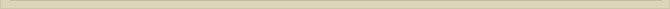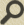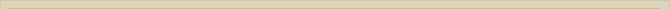## The 12 Ionization Levels of the Atom

When you are interested in physics you must read “Unbelievable“!

At the end of chapter 5. The Quantisation of Distance we stated that we would show that the rydberg-distance is exactly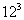*Rb and that there are 12 ionization levels.

Calculating the principal quantum number n for the rydberg-distance we found in accordance with QM that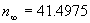. This means that according to QM there are over 40 ionization levels from the Bohr-distance to the rydberg-distance. The principal quantum number is calculated with the help of equation (7):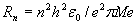Substitution of the Compton-radius (equation 13) gives

It is relevant to observe that with equation (7a) and therefore also with equation (7) we are calculating the ratio between Rn and Rc; we are comparing 3rd QD Rn with 2nd QD Rc.

With equation (6)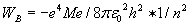we are with Me (and therefore Rc) in the 2nd QD. Equation (6) has therefore to be translated to the 3rd QD. The energy value of (6) is independent of the quantum dimension and therefore correct, but the calculated principal quantum number is not. We can achieve the correct transformation of equation (6) from 2-QD to 3-QD with (5c):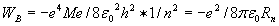For n=1 we calculate the Bohr-distance. We define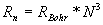instead of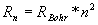in equation (9):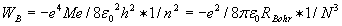Both equations are still identical for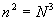.

Actually nothing has changed. The only difference is that N3 indicates that N is determined the 3rd quantum dimension and not the 2nd QD. All that changes is that N is not integer for all integer values of n. This is of no importance because the quantisation of distance has not changed. The ratio Rn/Rb is still integer related according to. The dimension correction only changed the number of ionization levels in the range from the Bohr-radius to the rydberg-distance from 41.5 to 12; the actual observed number of ionization levels.

At the Bohr-distance point-volumes in space around a charge +Q creates two “perfect” bulb shell tunnels with radius Rc (beginning of the 3rd quantum dimension). Outside the tunnels at the Bohr-radius space is not yet “perfectly” 3-dimensional for the electron.

For distances from +Q further than the rydbergconstant (N>12) there is no ionization level anymore because the 4th quantum dimension has started where space is everywhere “perfectly” 3-dimensional for the electron.

Next chapter: Planck’s constant